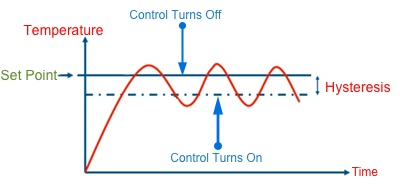# 简单阈值控制算法

## 概述## 代码

//Parameters
const int aihPin  = A0;
const int aihMargin  = 2;
const int aihThresh  = 306;

//Variables
int aihVal  = 0;
bool aihHystOn  = 0;

void setup() {
//Init Serial USB
Serial.begin(9600);
Serial.println(F("Initialize System"));
//Init AnalogHyst
pinMode(aihPin, INPUT);
}

void loop() {
hystThresh();
delay(200);
}

////Test routine for AnalogHyst
Serial.print(F("aih val ")); Serial.println(aihVal);
}

void hystThresh( ) { /* function hystThresh */

if (aihVal >= (aihThresh + aihMargin)) {
aihHystOn = true;
}
if (aihVal <= (aihThresh - aihMargin)) {
aihHystOn = false;
}

Serial.print(F("aih hist : ")); Serial.println(aihHystOn);
}